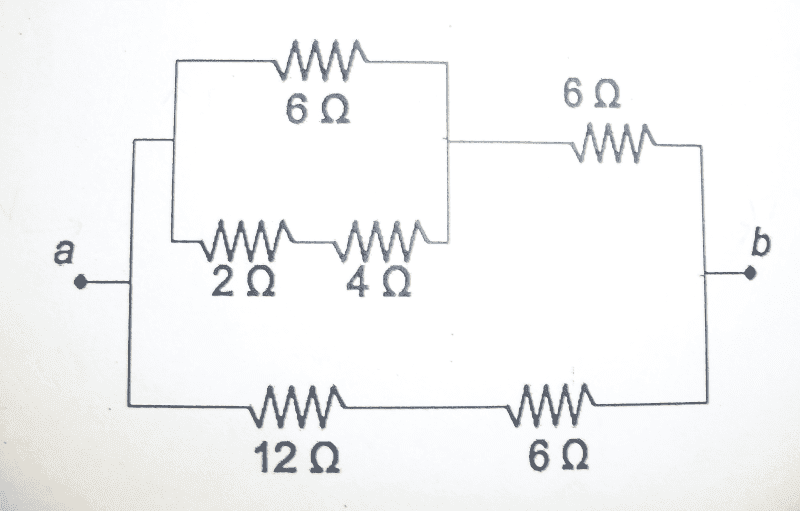# : Finding the Current and Power across each Resistor in a Complicated Network

sweetdion
URGENT: Finding the Current and Power across each Resistor in a Complicated Network

## Homework Statement

For the circuit below, a 12 V batter is connected across the points a and b. Determine the following:

a) the equivalent resistance between points a and b
b) the total current flowing through the resistor network
c) the total power dissipated by the resistor network
d) the current through each individual resistor and the power dissipated by each resistor. Sum up the power dissipation from each and compare that sum to your result from c. Is this what you expect?## Homework Equations

series: Req=R1+R2+...
parallel: 1/Req=1/R1+1/R2+.....
I=V/R
P=I^2R

## The Attempt at a Solution

a) I know how to do this part. I get 6 ohms.
b) using I=V/R=12/6=2A
c) P=I^2R=2^2*6=24 Watts
d) I have no idea how to do this part. How does the Voltage split up? Does 6V go into the top part then the 6 splits in to 3 for each part within the part? Then combine to form 6 again once it comes out the square in the top part?

willem2

There's 12 across the top part and 12 V across the bottom part as well.
Find the equivalent resistance of the top and the bottom part and then
calculate the currents through both parts.

sweetdion

for top Req=9 ohms
bottom Req=18 ohms

top (using V=IR) I=12/9=4/3 Amps
bottom I=18/9=2 Amps

now how do i find the currents in each resistor?

johnnyies

the best way to do a problem like this is to add up all the resistors and redraw the circuit each time you combined two resistors. This way, once you have the final resistance, you can find the total current, total voltage, total power, etc. then you can work your way backwards by using the circuits you drew.

Remember that current is the same for resistors in series and voltage is the same for resistors in parallel

know your concepts, it will make problems like these 100% easier.

sweetdion

the best way to do a problem like this is to add up all the resistors and redraw the circuit each time you combined two resistors. This way, once you have the final resistance, you can find the total current, total voltage, total power, etc. then you can work your way backwards by using the circuits you drew.

Remember that current is the same for resistors in series and voltage is the same for resistors in parallel

know your concepts, it will make problems like these 100% easier.

I did as you suggested. The only part that's confusing me is the box on the top part of the circuit. with the 6, 2, and 4 ohm resistors. Since they are in parallel, the top and bottom both have 12 Volts going through them. So (using V=IR) does that mean there are 2 Amps going through the 6 ohm resistor. And 6 and 3 amps going through the 2 and 4 ohm resistors respectively?